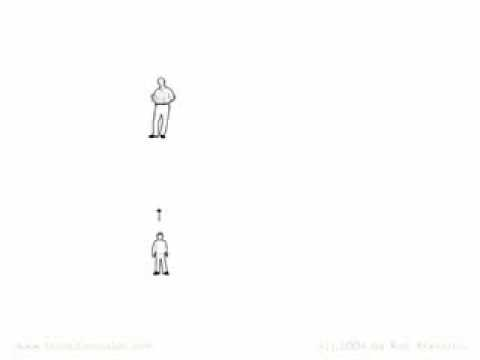# How many dimensions are there in all and what is each one?

I was reading a book and I saw the dimensions and I know that the first dimension is a line the second is a square and the third is a cube, but what are the other dimensions?

Relevance

According to Einstein the fourth one is space-time.

According to string theory there are 10 dimensions!

Nobody knows for sure.

• The basic 3: X, Y, Z and the fourth, t.

Horizontal, Vertical, Depth, time.

Previous post mentioned 10 dimensions explained here: http://www.youtube.com/watch?v=8Q_GQqUg6Ts• Um, it depends on what you mean.

You question isn't so simple.

Generally in the real world we count four.

But in mathematics it is easy to create spaces with as many dimensions as you want.